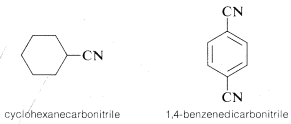# 7.9: Nitriles, RCN

$$\newcommand{\vecs}{\overset { \rightharpoonup} {\mathbf{#1}} }$$ $$\newcommand{\vecd}{\overset{-\!-\!\rightharpoonup}{\vphantom{a}\smash {#1}}}$$$$\newcommand{\id}{\mathrm{id}}$$ $$\newcommand{\Span}{\mathrm{span}}$$ $$\newcommand{\kernel}{\mathrm{null}\,}$$ $$\newcommand{\range}{\mathrm{range}\,}$$ $$\newcommand{\RealPart}{\mathrm{Re}}$$ $$\newcommand{\ImaginaryPart}{\mathrm{Im}}$$ $$\newcommand{\Argument}{\mathrm{Arg}}$$ $$\newcommand{\norm}{\| #1 \|}$$ $$\newcommand{\inner}{\langle #1, #2 \rangle}$$ $$\newcommand{\Span}{\mathrm{span}}$$ $$\newcommand{\id}{\mathrm{id}}$$ $$\newcommand{\Span}{\mathrm{span}}$$ $$\newcommand{\kernel}{\mathrm{null}\,}$$ $$\newcommand{\range}{\mathrm{range}\,}$$ $$\newcommand{\RealPart}{\mathrm{Re}}$$ $$\newcommand{\ImaginaryPart}{\mathrm{Im}}$$ $$\newcommand{\Argument}{\mathrm{Arg}}$$ $$\newcommand{\norm}{\| #1 \|}$$ $$\newcommand{\inner}{\langle #1, #2 \rangle}$$ $$\newcommand{\Span}{\mathrm{span}}$$$$\newcommand{\AA}{\unicode[.8,0]{x212B}}$$

1. Compounds with the $$\equiv N$$ function are named by adding the suffix nitrile to the main-chain hydrocarbon that includes the carbon linked to the nitrile ($$\equiv N$$) function. The chain is numbered so the $$CN$$ carbon is $$C1$$:2. Compounds of the type $$RCN$$ have to be called carbonitriles when $$R$$ is a cycloalkane or similar group:3. Nitriles can be regarded as derivatives of carboxylic acids because the acid, $$RCO_2H$$, usually can be obtained from the nitrile, $$RCN$$:A common system of naming nitriles takes the name of the corresponding carboxylic acid and changes the suffix -oic to -onitrile:4. The substituent name for $$-CN$$ is cyano. For example,